# Determine whether the differential equation is linear or nonlinear Problems For Problems 1-6, determine whether the...

Determine whether the differential equation is linear or nonlinear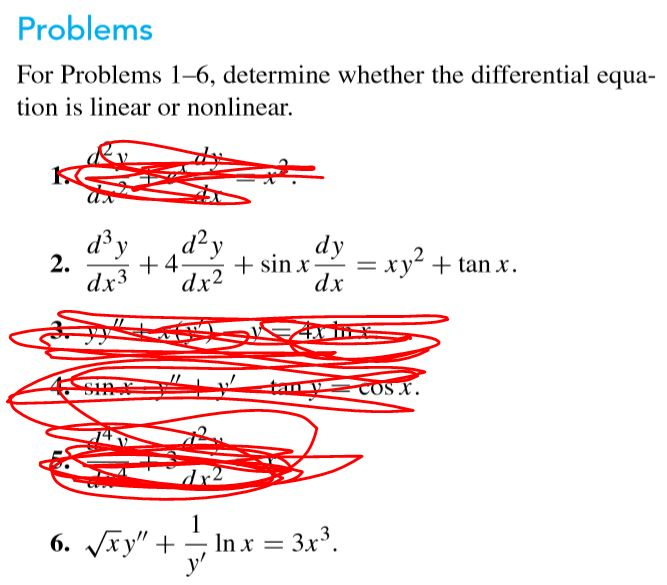Problems For Problems 1-6, determine whether the differential equa- tion is linear or nonlinear. d3 y day +4 2. dy + sin x dx = xy2 + + tan x dx3 dx2 COS X. 1 6. Vxy" + '++. In x = 3x3.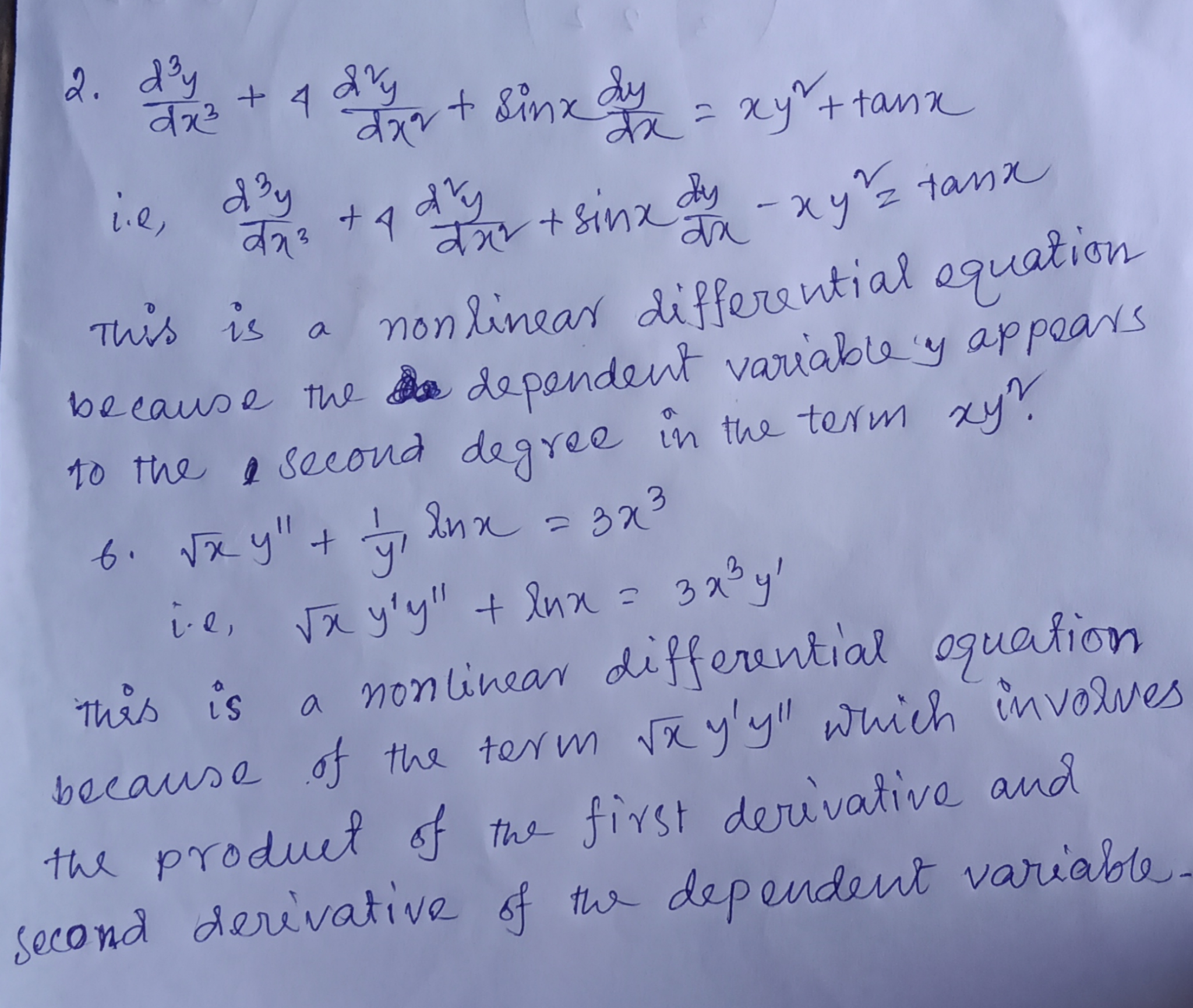##### Add Answer of: Determine whether the differential equation is linear or nonlinear Problems For Problems 1-6, determine whether the...
Similar Homework Help Questions
• ### 1. Determine the solution to the following differential equation (implicit if necessary): 2. Determine the general...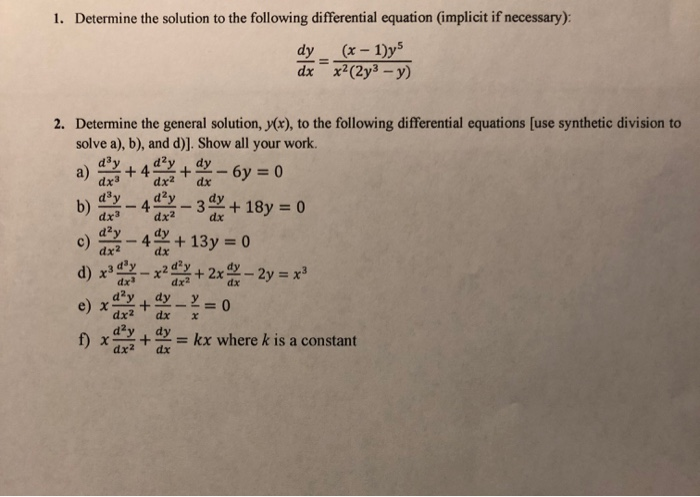1. Determine the solution to the following differential equation (implicit if necessary): 2. Determine the general solution, y(x), to the following differential equations [use synthetic division to solve a), b), and d)]. Show all your work dx3dx2 dx b)@y-4ーー3을y+18y = 0 d2 dx2 dx3 dx dx2 dx + 2-10 dy, dy _ y = 0 dx dx x f) χ +dy=kx where k is a constant dx2 dx

• ### 1: Determine whether the given differential equation is exact Q1: Determine whether the given differential equation...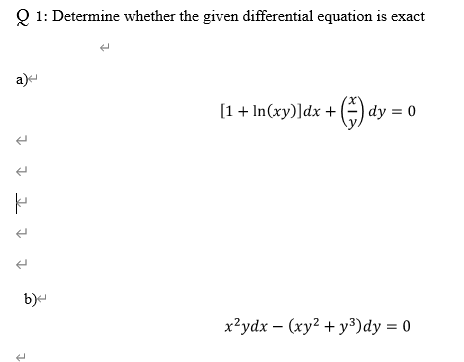1: Determine whether the given differential equation is exact Q1: Determine whether the given differential equation is exact a) [1 + In(xy)]dx + (dy = 0 소 소 전 소 소 be xydx - (xy2 + y3)dy = 0 t

• ### chapter 2 handout 14. help in diffeq question 1 or 2 please Homework Problems for Handout...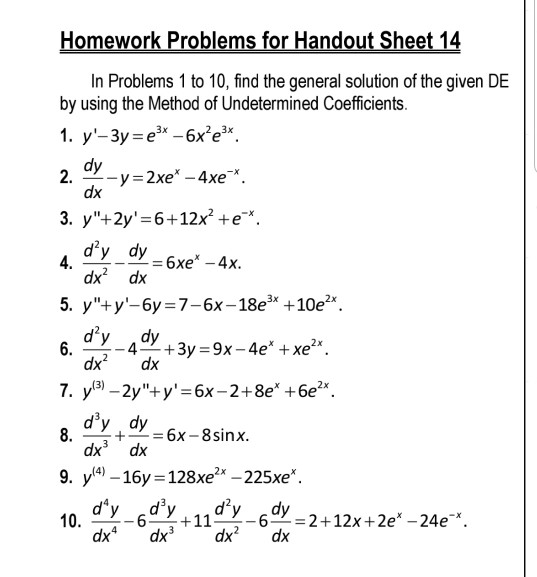chapter 2 handout 14. help in diffeq question 1 or 2 please Homework Problems for Handout Sheet 14 In Problems 1 to 10, find the general solution of the given DE by using the Method of Undetermined Coefficients 1. y-3y e-6xe3 dy --y = 2xe* -4xe 2. dx 3. y"+2y' 6+12x2 +e* d'y dy 6xe' -4x 4. dx2 dx 5. y"y'-6y 7-6x-18e3 +10e2x dy dy -4+3y 9x -4e xe2x. dx 6 dx2 7. y3 -2y"y' = 6x-2+8e* +6e2 d'y dy6x-8...

• ### Why isn't #dy/dx = 3x + 2y# a linear differential equation?

I've learned that linear differential equations are written in the form #dy/dx + P(x)y = Q(x)#. If you rewrote #dy/dx = 3x + 2y# as #dy/dx - 2y = 3x#, wouldn't your #P(x)# be #-2# and your #Q(x)# be #3x#, making this a linear differential equation? My teacher told me, however, that this is a nonlinear differential equation.

• ### 1. Identify each of the following equations as linear or nonlinear and also determine their order...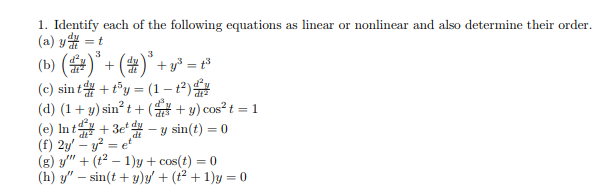1. Identify each of the following equations as linear or nonlinear and also determine their order dy (a) y = t 3 3 (b) ( dy + (c) sin t (d) (1y) sin t ( cos2 t 1 y sin(t)= 0 (e) Int +3etdy dt (f) 2y'-y2 =e (g) y"(t2 1)y+cos(t (h) y"sin(ty)y(t21)y 0 = 0 1. Identify each of the following equations as linear or nonlinear and also determine their order dy (a) y = t 3 3 (b)...

• ### (1 point) Solve the following differential equation: (tan(x) 8 sin(x) sin(y))dx + 8 cos(2) cos(y)dy =...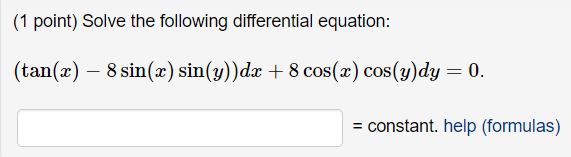(1 point) Solve the following differential equation: (tan(x) 8 sin(x) sin(y))dx + 8 cos(2) cos(y)dy = 0. = constant. help (formulas)

• ### 1) Solve The Differential Equation: a) d3y ,d2y dy -y 0 dx dx3 3 3 b)...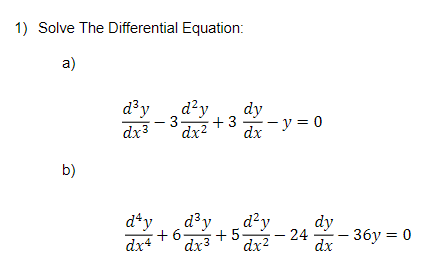1) Solve The Differential Equation: a) d3y ,d2y dy -y 0 dx dx3 3 3 b) dy 6 dx4 ,d2y 5 dx224 dy 36y 0 dx dx3

• ### Solve the first-order linear differential equation.

Solve the first-order linear differential equation.y' + 6xy = 36x and (y - 49)sin(x) dx - dy = 0

• ### linear differential equation

Solve the linear differential equationdydx=2 - (x +6) yx + 5,y(0) = 6Any help will be much appreciated :)

• ### Differential equation

Solve the following differential equation:(sin( y ) +3 cos( x ) ) dx + (2 y + x cos(y)) dy =0Thankyou!

Free Homework App